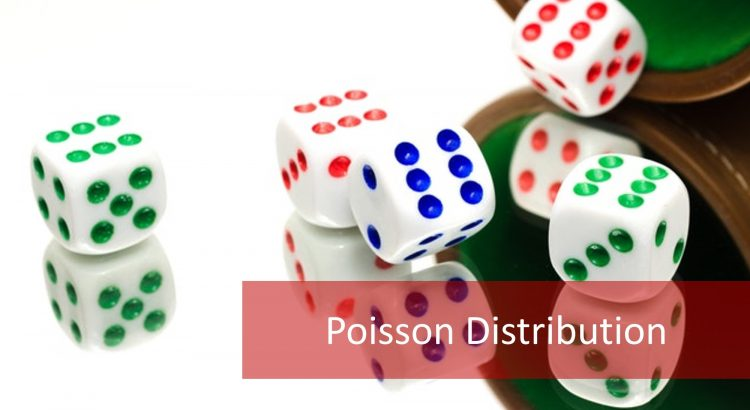How to Calculate Probability Using the Poisson Distribution?

Lean Six Sigma Green Belt course graduates deal with two types of data during the Six Sigma Measure phase of their Six Sigma DMAIC projects: continuous data and discrete data. The Poisson distribution is a probability distribution for discrete data which takes on the values which are X = 0, 1, 2, 3 and so […]